Question

# A compound of molar mass 166 contains only carbon, hydrogen, oxygen, and bromine. Analysis shows that...

A compound of molar mass 166 contains only carbon, hydrogen, oxygen, and bromine. Analysis shows that a sample of the compound contains six times as much carbon as hydrogen, by mass.

Part A

Calculate the molecular formula of the compound.

Express your answer as a chemical formula. Enter the elements in the order: C, H, O, Br.

C6H7O2Br5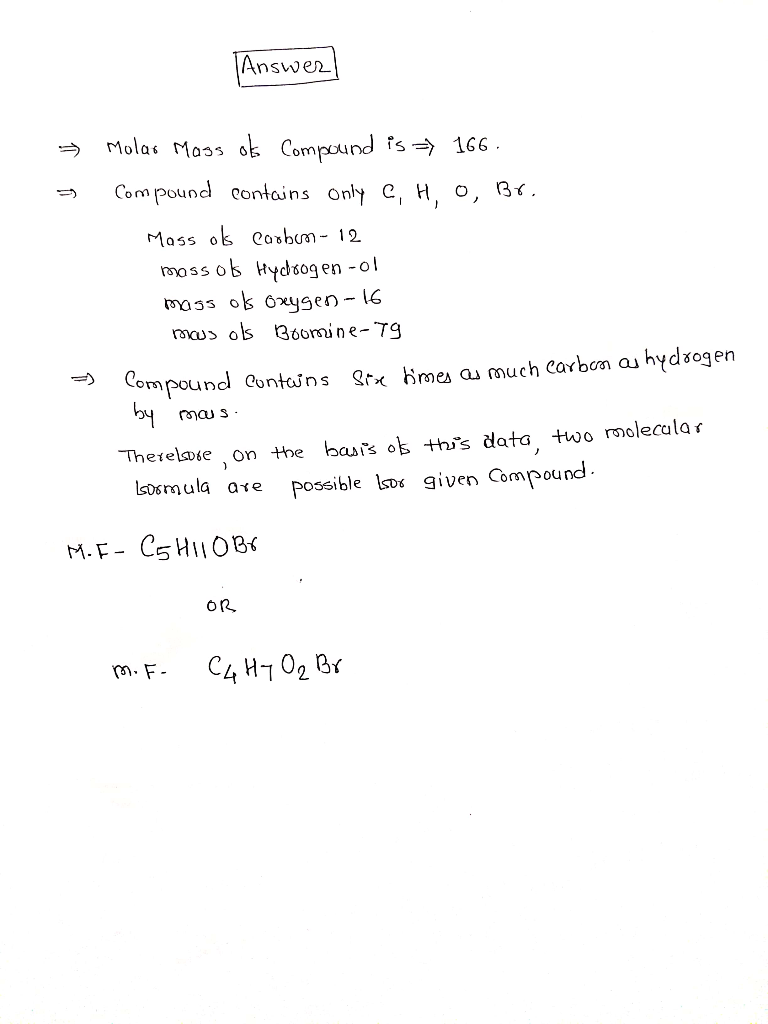#### Earn Coins

Coins can be redeemed for fabulous gifts.

Similar Homework Help Questions
• ### A compound contains only carbon, hydrogen, and oxygen. Combustion of 10.68 mg of the compound yields...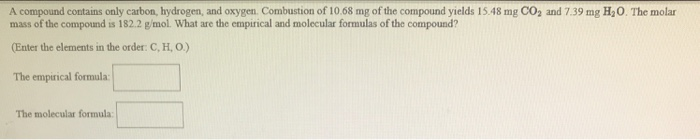A compound contains only carbon, hydrogen, and oxygen. Combustion of 10.68 mg of the compound yields 15.48 mg CO2 and 7.39 mg H20. The molar mass of the compound is 182.2 g/mol What are the empirical and molecular formulas of the compound? (Enter the elements in the order: C, H, O) The empirical formula: The molecular formula

• ### Combustion analysis 12.01 g of an unknown sample, which contains only carbon, hydrogen and oxygen, produced...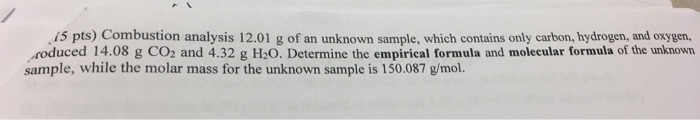Combustion analysis 12.01 g of an unknown sample, which contains only carbon, hydrogen and oxygen, produced 14.08 g co2 and 4.32 h2o. Determine the empirical formula and molecular formula of the unknown sample, while the molar mass for the unknown sample is 150.078 g/mol. 15 pts) Combustion analysis 12.01 g of an unknown sample, which contains only carbon, hydrogen, and oxygen, roduced 14.08 g CO2 and 4.32 g H20. Determine the empirical formula and molecular formula of the unknown sample,...

• ### 5. 74.1% carbon, 8.6% hydrogen, & 17.3% nitrogen by mass. Its molar mass = 160 g/mol....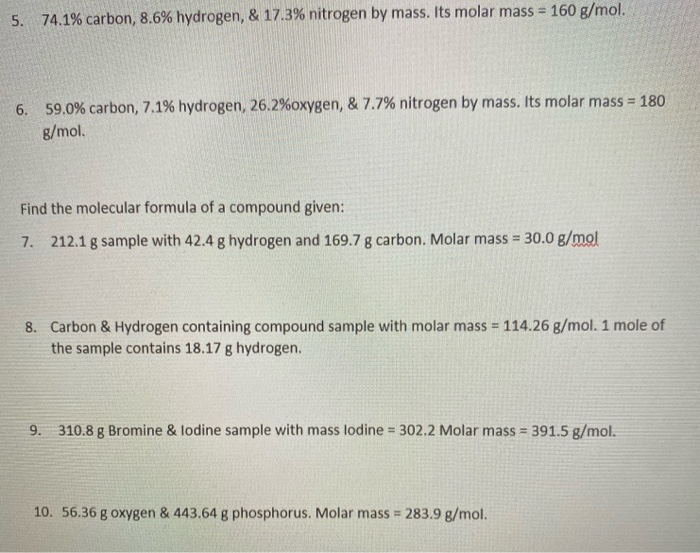5. 74.1% carbon, 8.6% hydrogen, & 17.3% nitrogen by mass. Its molar mass = 160 g/mol. 6. 59.0% carbon, 7.1% hydrogen, 26.2%oxygen, & 7.7% nitrogen by mass. Its molar mass = 180 g/mol. Find the molecular formula of a compound given: 7. 212.1 g sample with 42.4 g hydrogen and 169.7 g carbon. Molar mass = 30.0 g/mol 8. Carbon & Hydrogen containing compound sample with molar mass = 114.26 g/mol. 1 mole of the sample contains 18.17 g hydrogen....

• ### A compound contains only carbon, hydrogen, and oxygen. Combustion of 18.92 g of the compound yields 27.73 g of CO2 and...

A compound contains only carbon, hydrogen, and oxygen. Combustion of 18.92 g of the compound yields 27.73 g of CO2 and 11.35 g of H2O. The molar mass of the compound is 90.078 g/mol. *Each part of this problem should be submitted separately to avoid losing your work* 1. Calculate the grams of carbon (C) in 18.92 g of the compound: grams 2. Calculate the grams of hydrogen (H) in 18.92 g of the compound. grams 3. Calculate the grams...

• ### 5. (12 pts) A compound contains only carbon, hydrogen, and oxygen. If complete combustion of 70.86...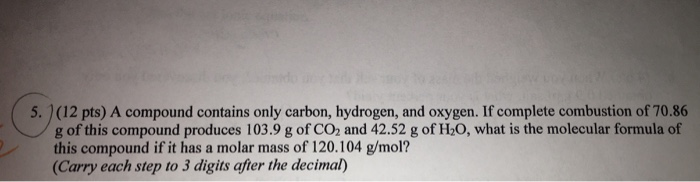5. (12 pts) A compound contains only carbon, hydrogen, and oxygen. If complete combustion of 70.86 g of this compound produces 103.9 g of CO2 and 42.52 g of H20, what is the molecular formula of this compound if it has a molar mass of 120.104 g/mol? (Carry each step to 3 digits after the decimal)

• ### A compound that contains only carbon, hydrogen, and oxygen is 45.3 % C and 9.43 %...

A compound that contains only carbon, hydrogen, and oxygen is 45.3 % C and 9.43 % H by mass. What is the empirical formula of the substance? Enter the correct subscript for each atom using the smallest whole number. Its not C1H3O1

• ### A 25.121 mg sample of a chemical known to contain only carbon, hydrogen, sulfur, and oxygen...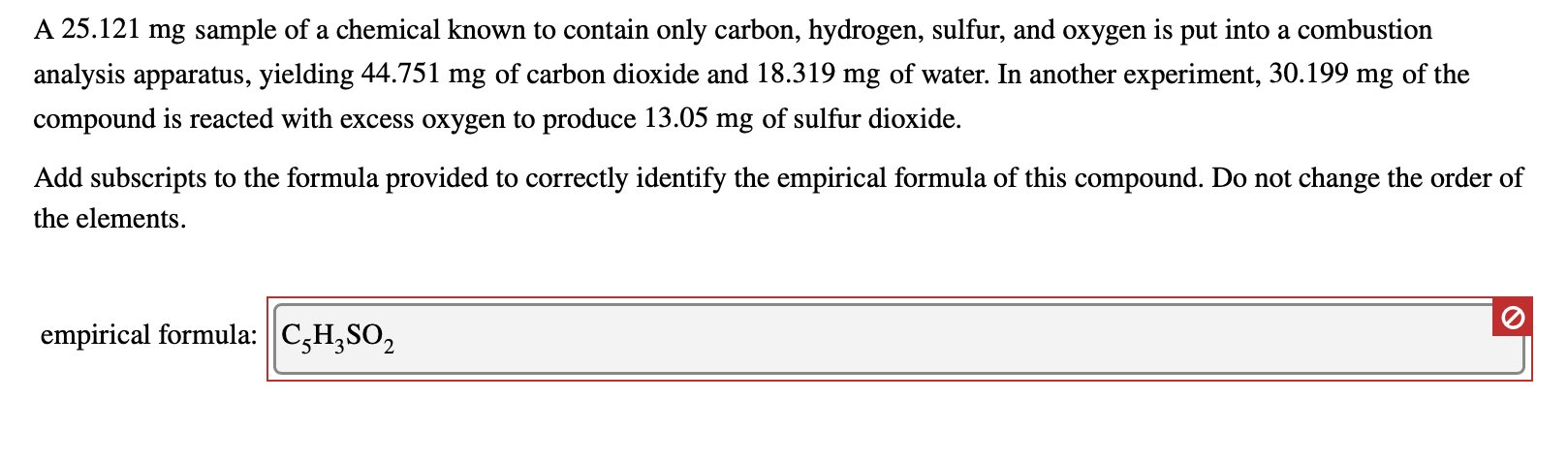A 25.121 mg sample of a chemical known to contain only carbon, hydrogen, sulfur, and oxygen is put into a combustion analysis apparatus, yielding 44.751 mg of carbon dioxide and 18.319 mg of water. In another experiment, 30.199 mg of the compound is reacted with excess oxygen to produce 13.05 mg of sulfur dioxide. Add subscripts to the formula provided to correctly identify the empirical formula of this compound. Do not change the order of the elements. A 25.121 mg...

• ### 1. Dioxybenzanone is a compound found in sunscreen, and only contains carbon, oxygen, and hydrogen. Analysis...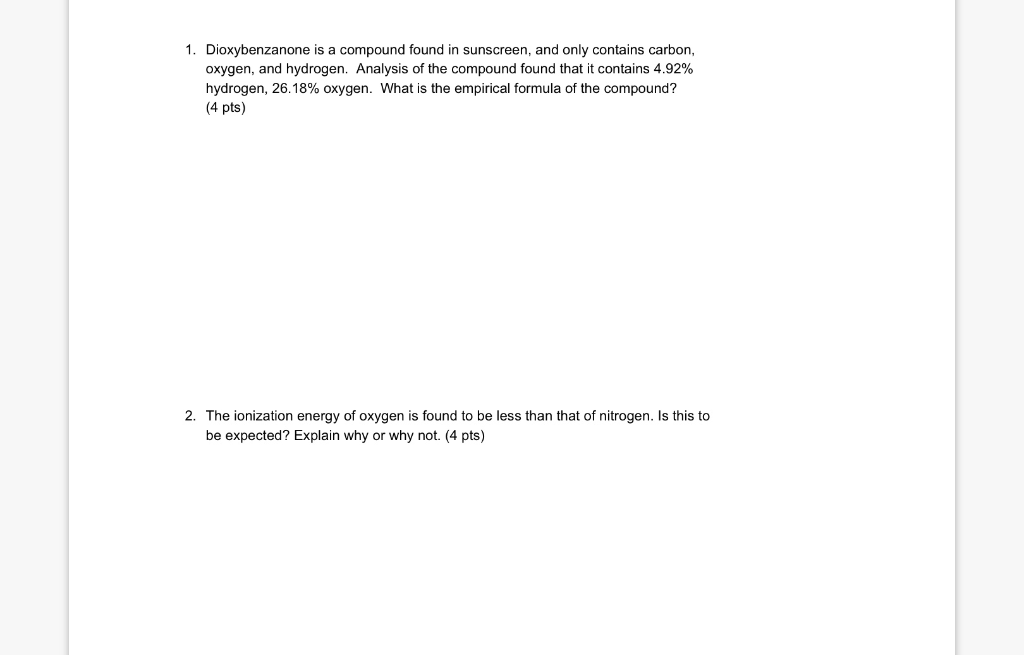1. Dioxybenzanone is a compound found in sunscreen, and only contains carbon, oxygen, and hydrogen. Analysis of the compound found that it contains 4.92% hydrogen, 26.18% oxygen. What is the empirical formula of the compound? (4 pts) 2. The ionization energy of oxygen is found to be less than that of nitrogen. Is this to be expected? Explain why or why not. (4 pts)

• ### 2:21 B 81% Analysis by mass of a compound containing carbon, hydrogen, oxygen and nitrogen only...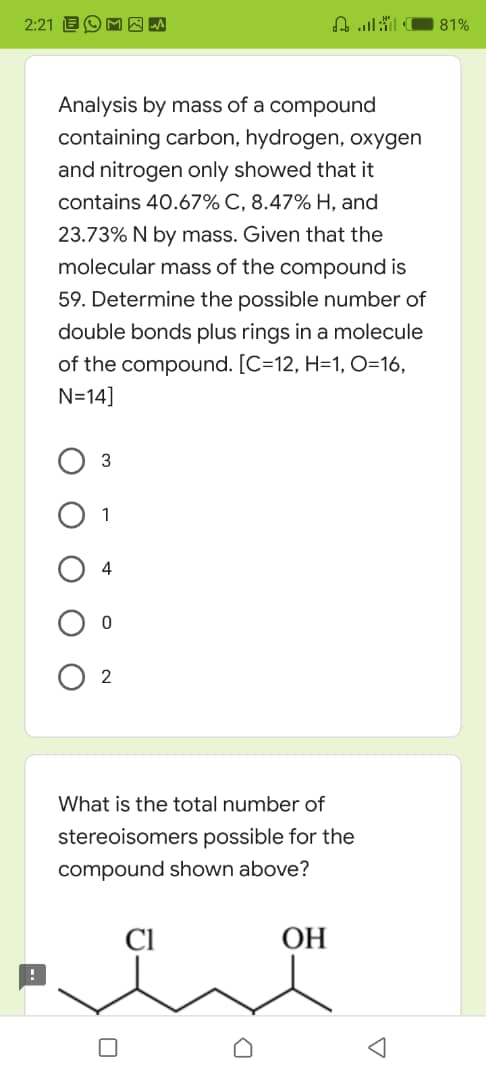2:21 B 81% Analysis by mass of a compound containing carbon, hydrogen, oxygen and nitrogen only showed that it contains 40.67% C, 8.47% H, and 23.73% N by mass. Given that the molecular mass of the compound is 59. Determine the possible number of double bonds plus rings in a molecule of the compound. [C=12, H=1, O=16, N=14] 3 1 4 0 2 What is the total number of stereoisomers possible for the compound shown above? OH w

• ### A compound composed of only hydrogen and oxygen is 5 94% hydrogen by mass. The molar mass of this compound is 34...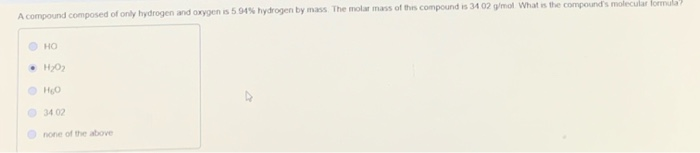A compound composed of only hydrogen and oxygen is 5 94% hydrogen by mass. The molar mass of this compound is 3402 g/mol What is the compound's molecular formula О но • H202 HO 3402 none of the above